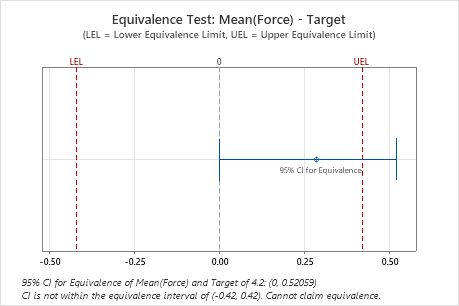# Difference for 1-Sample Equivalence Test

Find definitions and interpretation guidance for every measure that is provided in the Difference table of the 1-sample equivalence test.

## Difference

The difference is the difference between the sample mean and the target.

### Interpretation

The difference of your sample data provides an estimate of the difference between the population mean and the target.

Because the difference is based on sample data and not on the entire population, you cannot be certain that it equals the difference between the population mean and the target. To assess the precision of the estimate for the population, you can use a confidence interval.

## SE

The standard error of the difference (SE) estimates the variability of the difference between the target and the sample mean that you would obtain if you took repeated samples from the same population. The standard error of the difference estimates the variability between samples, whereas the standard deviation measures the variability within a single sample.

### Interpretation

Use the standard error of the difference to determine how precisely the difference between the sample mean and the target estimates the difference between the population mean and the target.

Lower values of the standard error indicate a more precise estimate. Usually, a larger standard deviation results in a larger standard error of the difference and a less precise estimate. A larger sample size results in a smaller standard error of the difference and a more precise estimate.

Minitab uses the standard error of the difference to calculate the test statistic (t-value).

## Confidence interval (CI)

The confidence interval provides a range of likely values for the difference between the population mean and the target. The lower confidence limit defines a value that the difference is likely to be greater than. The upper confidence limit defines a value that the difference is likely to be less than.

###### Note

If you perform an equivalence test using the default settings, Minitab displays a confidence interval for equivalence. If you change the default setting and use the alternate method for calculating the confidence interval, Minitab displays a standard confidence interval. For information on the difference between these intervals, go to Confidence intervals in equivalence testing.

### Interpretation

Compare the confidence interval with the equivalence limits. If the confidence interval is completely within the equivalence limits, you can claim that the population mean is equivalent to the target. If part of the confidence interval is outside the equivalence limits, you cannot claim equivalence.In these results, the 95% confidence interval exceeds the upper equivalence limit. Therefore, you cannot claim that the population mean is equivalent to the target.

## Lower bound

Minitab displays a lower confidence bound if you select an alternative hypothesis that includes only a lower limit for the difference. The lower bound indicates a value that the difference between the population mean and the target is likely to be greater than.

### Interpretation

Use the lower confidence bound to determine whether the difference between the population mean and the target is greater than the lower limit. If the lower confidence bound is greater than the lower limit, you can claim that the difference between the population mean and the target is greater than the lower limit.

## Upper bound

Minitab displays an upper confidence bound if you select an alternative hypothesis that includes only an upper limit for the difference. The upper bound indicates a value that the difference between the population mean and the target is likely to be less than.

### Interpretation

Use the upper confidence bound to determine whether the difference between the population mean and the target is less than the upper limit. If the upper confidence bound is less than the upper limit, you can claim that the difference between the population mean and the target is less than the upper limit.

## Equivalence interval

An equivalence interval is a range of values that are close enough to the target to be considered equivalent. The interval is based on your knowledge of the product or process and is defined by a lower equivalence limit (LEL) and an upper equivalence limit (UEL).

### Interpretation

Compare the confidence interval with the equivalence limits. If the confidence interval is completely within the equivalence limits, you can claim that the population mean is equivalent to the target. If part of the confidence interval is outside the equivalence limits, you cannot claim equivalence.In these results, the 95% confidence interval exceeds the upper equivalence limit. Therefore, you cannot claim that the population mean is equivalent to the target.

## Lower limit

The lower limit of acceptability for the difference between the mean and the target. You want the difference between the mean of the test population and the target to be greater than the lower limit.

### Interpretation

Compare the lower limit with the lower confidence bound. If the lower confidence bound is greater than the lower limit, you can claim that the difference between the population mean and the target is greater than the lower limit.

## Upper limit

The upper limit of acceptability for the difference between the mean and the target. You want the difference between the mean of the test population and the target to be less than the upper limit.

### Interpretation

Compare the upper limit with the upper confidence bound. If the upper confidence bound is less than the upper limit, you can claim that the difference between the population mean and the target is less than the upper limit.

By using this site you agree to the use of cookies for analytics and personalized content.  Read our policy